A mixture of calculator and non-calculator skills linking functions with graphs.

Syllabus content: SL 2.2, SL 2.5, SL 2.6, SL 2.7

I have made 5 worksheets and 4 treasure hunts to help with this topic. They are:

1. Basic functions
5. Bridge building
6. Treasure hunt: discriminant of quadratics
7. Treasure hunt: functions domains and ranges
8. Treasure hunt: graphing skills

You can scroll down below to see the images of the questions.  You can download both the questions and mark scheme from the bottom of the page.

1. Basic functionsNote:  This is a short worksheet which can be used in lesson or for homework.  It covers syllabus point: SL 2.2 and SL 2.5.  Students explore domains and ranges and function notation as well as inverses.Note:  This is a short worksheet which can be used in lesson or for homework.  It covers syllabus point: SL 2.6.  Students work with quadratic equations and sketch graphs.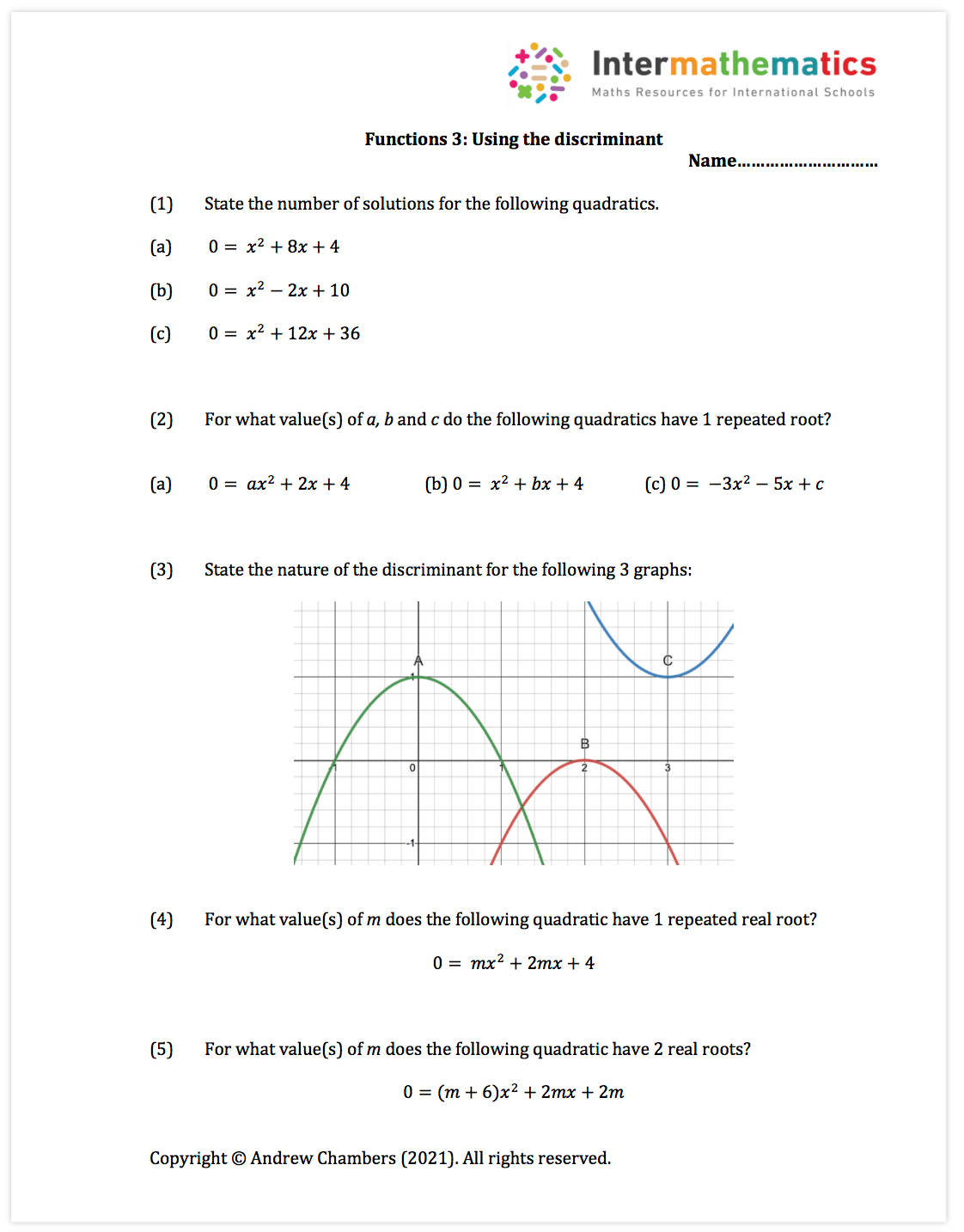Note:  This is a short worksheet which can be used in lesson or for homework.  It covers syllabus point: SL 2.7.  Students work with the discriminant of quadratics to find the number of solutions.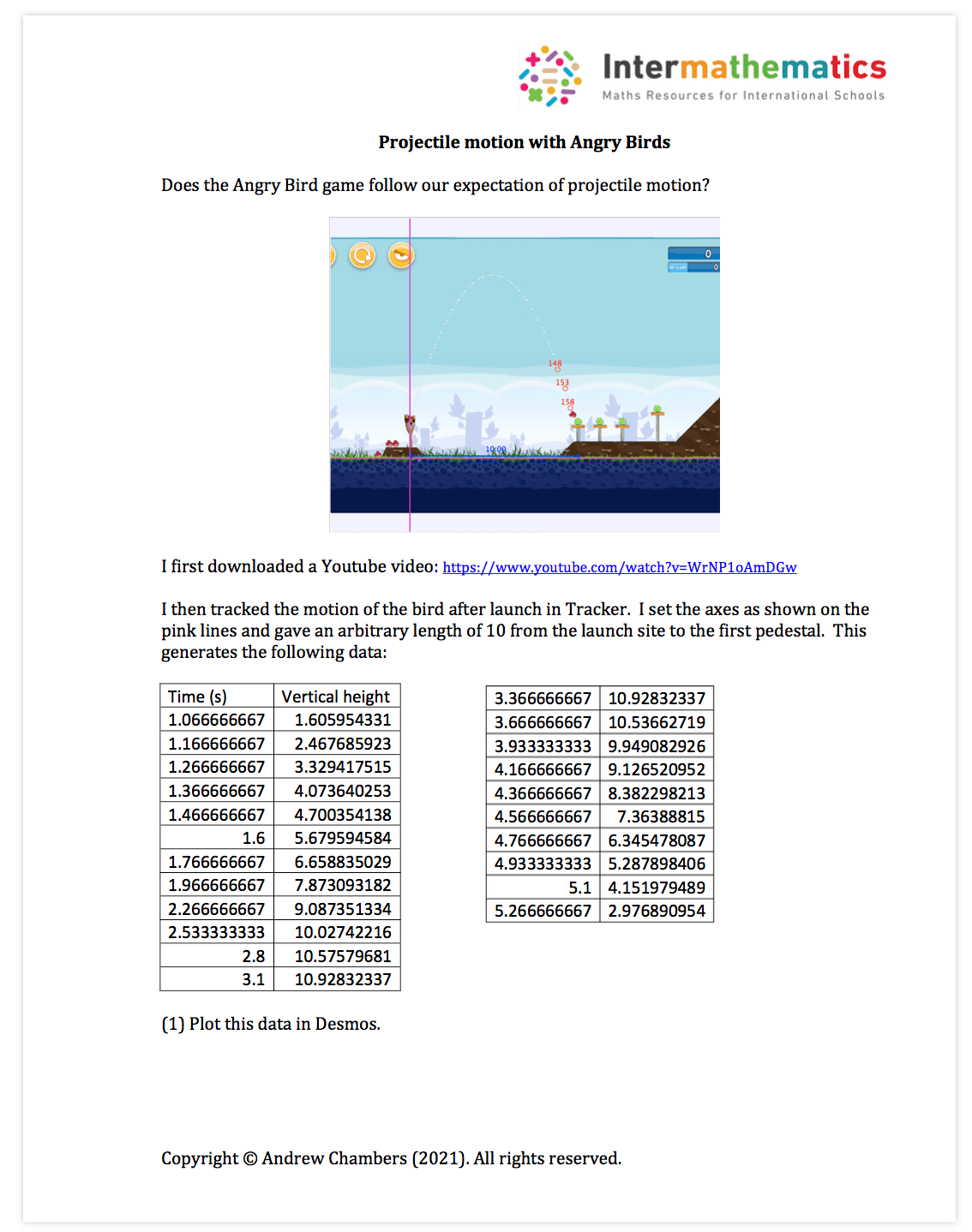Note:  This is an investigation idea which starts by tracking data from a video, and then uses regression on Desmos to model projectile motion.

5. Bridge Building: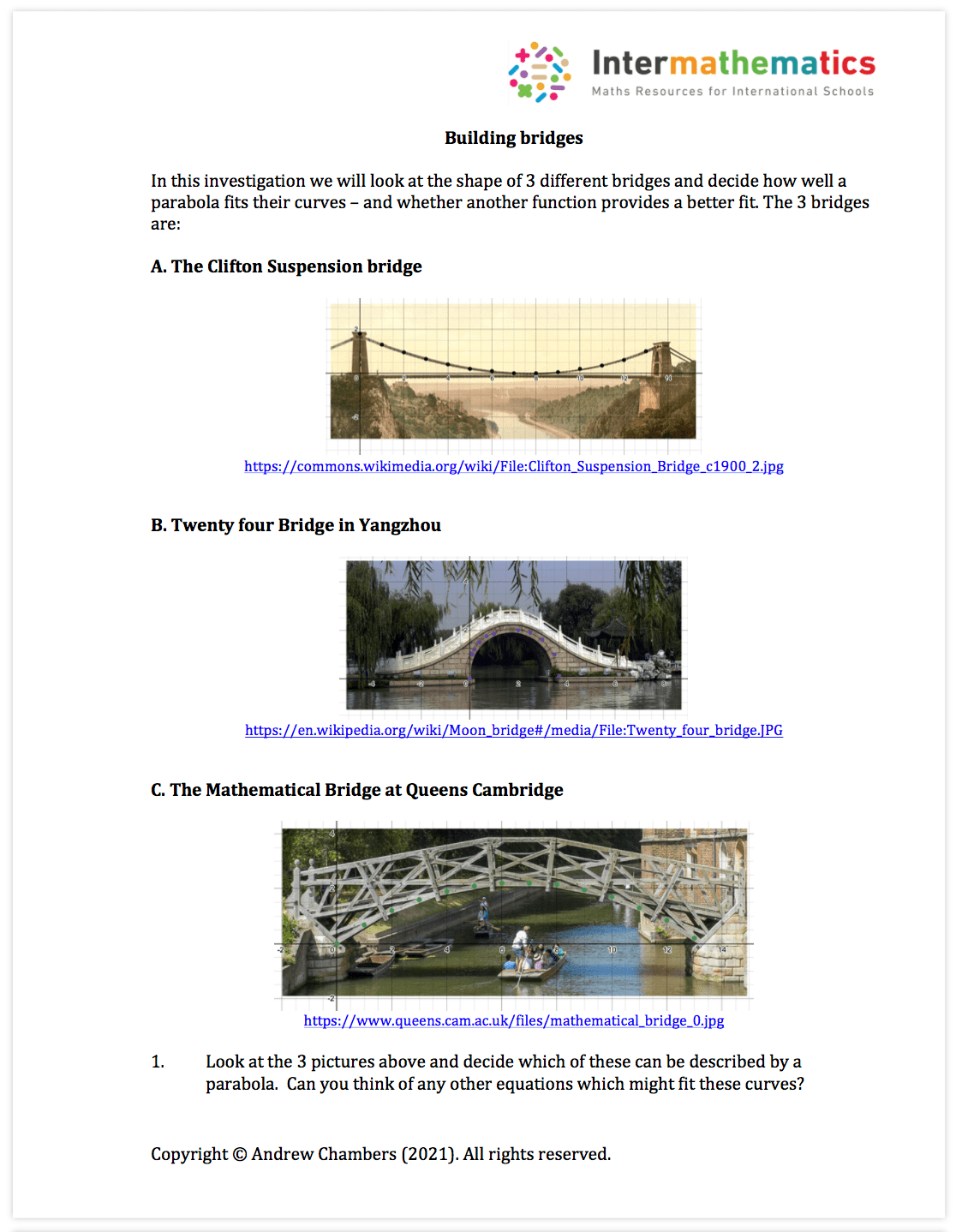Note:  This is an investigation idea which looks at the shapes of bridges and how closely they follow a quadratic curve.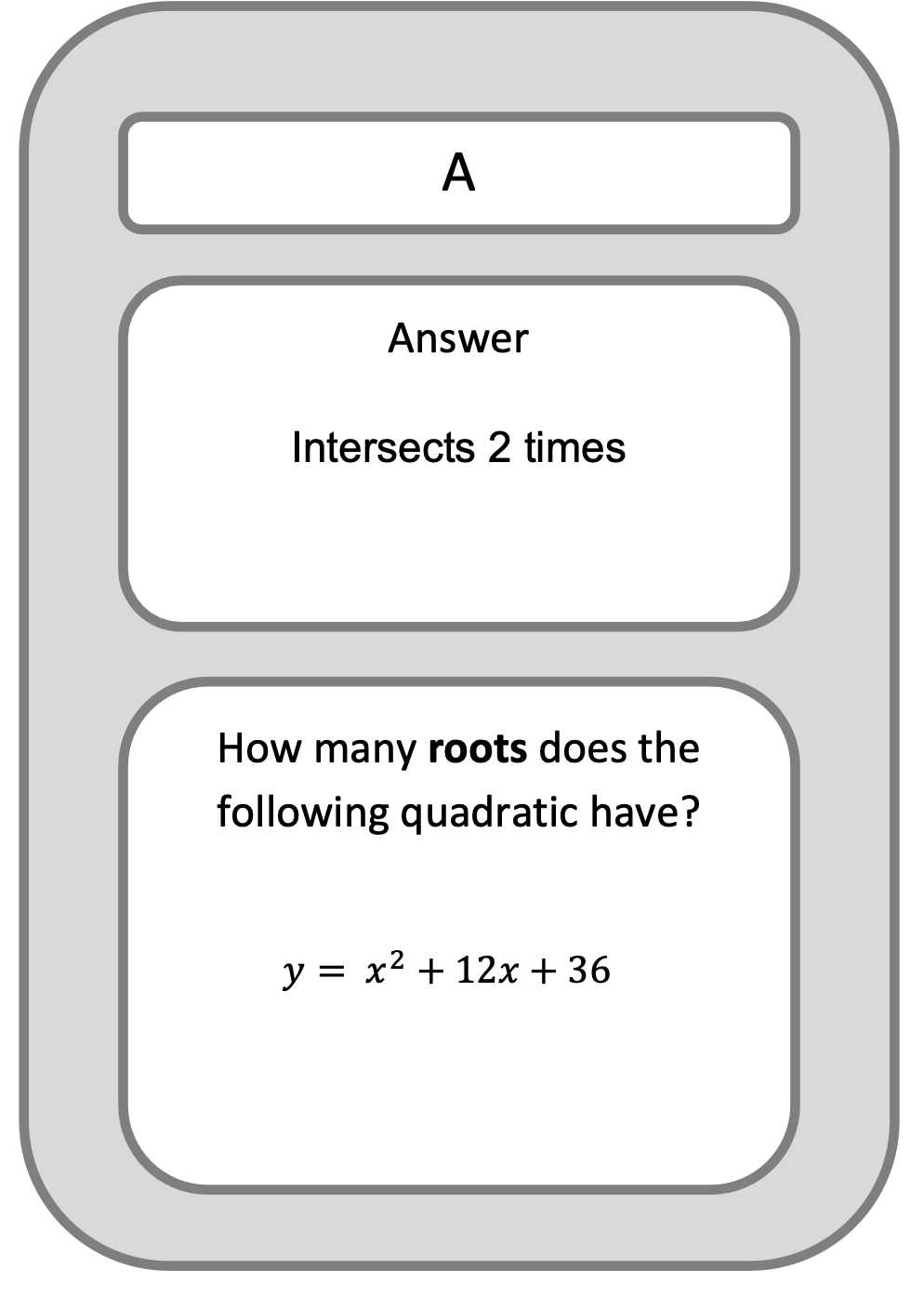Note:  This is an activity which can be done in pairs or as a whole class activity.  Students solve a mixture of discriminant problems.

Treasure hunt: Functions Domains and Ranges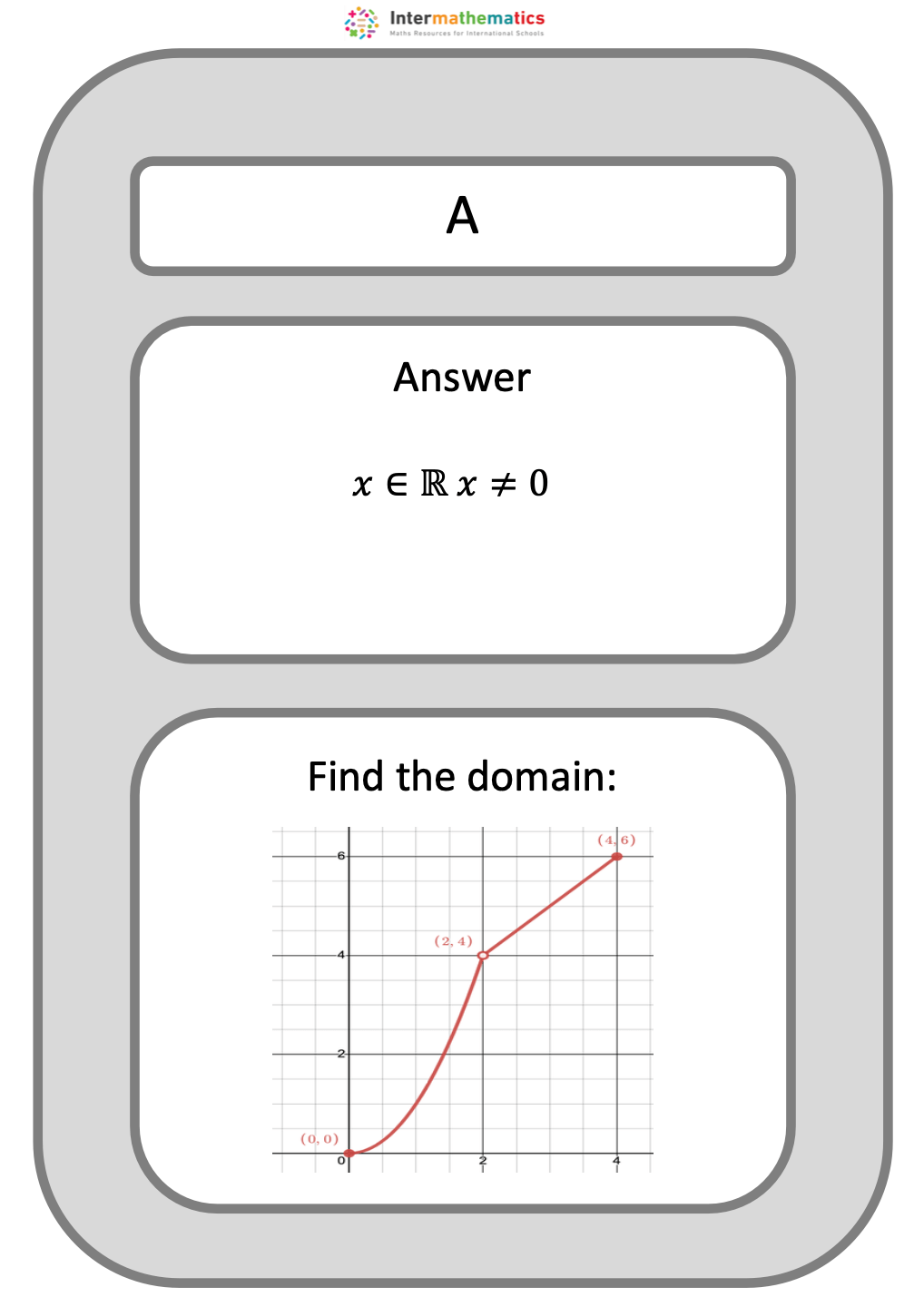Note:  This is an activity which can be done in pairs or as a whole class activity.  Students solve a mixture of problems around domain and range.

Treasure hunt: graphing skillsNote:  This is an activity which can be done in pairs or as a whole class activity.  Students solve a mixture of graph sketching problems.Note:  This is an activity which can be done in pairs or as a whole class activity.  Students solve a mixture of quadratic problems.